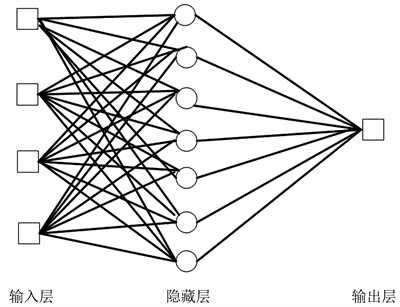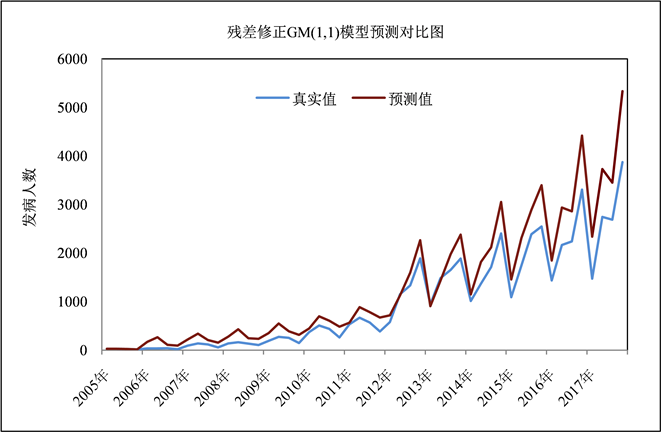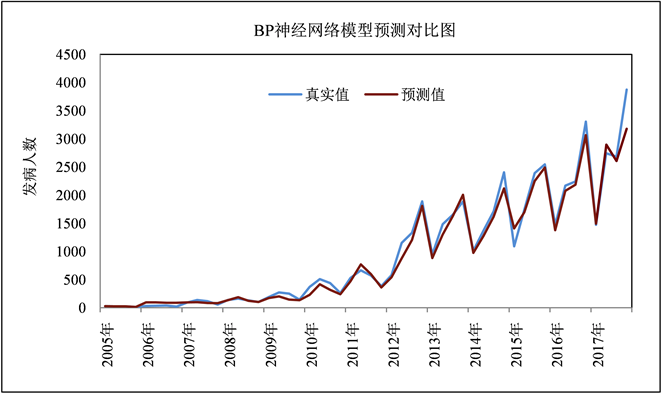﻿ 四川省艾滋病发病人数模型及预测

四川省艾滋病发病人数模型及预测Model and Prediction of AIDS Incidence in Sichuan Province

Abstract: In order to explore the trend of the number of AIDS cases in Sichuan province, this paper uses the residual correction GM(1,1) model and the BP (Back Propagation) neural network model to predict the number of cases and compare the prediction results. Based on the data on the number of AIDS cases in Sichuan Province from the first quarter of 2005 to the fourth quarter of 2016, a residual correction GM(1,1) model and a BP neural network model were established to predict the number of cases from the first quarter to the fourth quarter of 2017. The residual correction GM(1,1) model predicted that the average absolute error (MAE) and average absolute percentage error (MAPE) of the number of AIDS cases in Sichuan Province in 2017 were 1019 and 0.4023, respectively; the BP neural network model predicted that MAE and MAPE of the number of AIDS cases in Sichuan Province in 2017 were 236 and 0.0697, respectively. Compared with the residual correction GM(1,1) model, the BP neural network model can better fit the incidence trend of AIDS in Sichuan Province, therefore, it is more suitable for short-term prediction of AIDS incidence in Sichuan Province.

1. 引言

2. 研究方法与模型建立

2.1. 残差修正GM(1,1)模型

2.1.1. 建立GM(1,1)模型

GM(1,1)模型建立过程如下：

1) 构建原始数据序列：设原始数据序列为 ${y}^{\left(0\right)}=\left({y}^{\left(0\right)}\left(1\right),{y}^{\left(0\right)}\left(2\right),\cdots ,{y}^{\left(0\right)}\left(n\right)\right)$，对原始数据做一次累

2) 均值生成：对一次累加生成值 ${y}^{\left(1\right)}\left(k\right)$ 进行均值处理，得到数据序列： ${z}^{\left(1\right)}=\left({z}^{\left(1\right)}\left(2\right),{z}^{\left(1\right)}\left(3\right),\cdots ,{z}^{\left(1\right)}\left(n\right)\right)$，其中 ${z}^{\left(1\right)}\left(k\right)=\alpha {y}^{\left(1\right)}\left(k\right)+\left(1-\alpha \right){y}^{\left(1\right)}\left(k-1\right)$$k=2,3,\cdots ,n$$0\le \alpha \le 1$，通常可取 $\alpha =0.5$

3) 建立白化微分方程：建立灰色GM(1,1)白化形式的微分方程：

$\frac{\text{d}{y}^{\left(1\right)}}{\text{d}t}+a{y}^{\left(1\right)}=b$

$B=\left[\begin{array}{cc}-\frac{1}{2}{z}^{\left(1\right)}\left(2\right)& 1\\ -\frac{1}{2}{z}^{\left(1\right)}\left(3\right)& 1\\ ⋮& ⋮\\ -\frac{1}{2}{z}^{\left(1\right)}\left(n\right)& 1\end{array}\right]$ , ${M}_{n}=\left[\begin{array}{c}{y}^{\left(0\right)}\left(2\right)\\ {y}^{\left(0\right)}\left(3\right)\\ ⋮\\ {y}^{\left(0\right)}\left(n\right)\end{array}\right]$

$\left[\begin{array}{c}a\\ b\end{array}\right]={\left({B}^{T}B\right)}^{-1}{B}^{T}{M}_{n}$

4) 得出预测序列： ${\stackrel{^}{y}}^{\left(1\right)}=\left({\stackrel{^}{y}}^{\left(1\right)}\left(1\right),{\stackrel{^}{y}}^{\left(1\right)}\left(2\right),\cdots ,{\stackrel{^}{y}}^{\left(1\right)}\left(n\right)\right)$，其中：

${\stackrel{^}{y}}^{\left(1\right)}\left(k\right)=\left[{y}^{\left(0\right)}\left(1\right)-\frac{b}{a}\right]{\text{e}}^{-a\left(k-1\right)}+\frac{b}{a}$ , $k=2,3,\cdots ,n$ ;

${\stackrel{^}{y}}^{\left(0\right)}\left(k\right)={\stackrel{^}{y}}^{\left(1\right)}\left(k\right)-{\stackrel{^}{y}}^{\left(1\right)}\left(k-1\right)=\left[{y}^{\left(0\right)}\left(1\right)-\frac{b}{a}\right]{\text{e}}^{-a\left(k-1\right)}\left(1-{\text{e}}^{a}\right)$ , $k=2,3,4,\cdots ,n$ .

2.1.2. 检验精度Table 1. Model accuracy comparison table

2.1.3. GM(1,1)模型的修正

${\stackrel{^}{\epsilon }}^{\left(0\right)}\left(k\right)={\stackrel{^}{\epsilon }}^{\left(1\right)}\left(k\right)-{\stackrel{^}{\epsilon }}^{\left(1\right)}\left(k-1\right)=\left[{\epsilon }^{\left(0\right)}\left(1\right)-\frac{b}{a}\right]{\text{e}}^{-a\left(k-1\right)}\left(1-{\text{e}}^{a}\right),k=2,3,4,\cdots ,n$

2.2. BP神经网络算法

BP神经网络(back-propagation neural network)是一种多层前馈的神经网络(见图1)，该网络的主要特点是信号向前传递，误差反向传递；在前向传递的过程中，需要输入的数据作为输入信号从输入层经过隐藏层逐层处理，到输出层输出；如果与真实输出信号存在误差，网络就转入误差反向传播过程，并根据误差大小来调整各层神经元之间的连接权值和偏倚。当误差达到预期时，网络学习的过程就会结束，之后得到调整后的输出值  。Figure 1. BP neural network structure diagram

2.3. 预测评价指标

$\text{MAE}=\frac{1}{n}\underset{k=1}{\overset{n}{\sum }}|{\stackrel{^}{y}}^{\left(0\right)}\left(k\right)-{y}^{\left(0\right)}\left(k\right)|$

$\text{MAPE}=\frac{100%}{n}\underset{k=1}{\overset{n}{\sum }}|\frac{{\stackrel{^}{y}}^{\left(0\right)}\left(k\right)-{y}^{\left(0\right)}\left(k\right)}{{y}^{\left(0\right)}\left(k\right)}|$

3. 实证研究分析

3.1. 数据来源

3.2. 残差修正GM(1,1)模型建立

3.2.1. GM(1,1)模型Table 2. Model-level evaluation of the number of patients in each quarter

3.2.2. 修正GM(1,1)模型

${\stackrel{^}{\epsilon }}^{\left(1\right)}\left(t\right)=-271.2337{\text{e}}^{0.214669\left(t-1\right)}+301.2337$

${\stackrel{^}{\epsilon }}^{\left(1\right)}\left(t\right)=-206.7147{\text{e}}^{0.2713\left(t-1\right)}+236.7147$Figure 2. Comparison of GM(1,1) model prediction

3.3. BP神经网络模型Table 3. Standardization of raw data in each quarterTable 4. Evaluation of the corresponding number of nodes in the hidden layer of the BP neural network modelFigure 3. Comparison of BP neural network model prediction

4. 模型预测结果比较Table 5. Comparison of prediction results between GM(1,1) and BP neural network (2017)

5. 讨论

 He, N., Zhang, J., Yao, J., Tian, X., Zhao, G., Jiang, Q. and Detels, R. (2009) Knowledge, Attitudes, and Practices of Voluntary HIV Counseling and Testing among Rural Migrants in Shanghai, China. AIDS Education & Prevention, 21, 560-5812.
https://doi.org/10.1521/aeap.2009.21.6.570

 戴色莺, 沈张伟, 范引光, 程晓莉, 叶冬青. 我国艾滋病预防控制中流行病学研究进展[J]. 中华疾病控制杂志, 2015, 19(12): 1282-1285.

 王楚雯, 胡颖, 侯颖. 广西壮族自治区艾滋病模型及预测分析[J]. 检验检疫学刊, 2020, 30(2): 6-9.

 彭志行, 陈峰. HIV/AIDS传播动力学模型研究进展[J]. 中国卫生统计, 2011, 28(6): 730-734.

 代玉巧, 严运楼, 刘政. 基于GM(1,1)模型的北京市卫生总费用及构成变化趋势预测分析[J]. 现代预防医学, 2021, 48(11): 1996-2000.

 程燕, 刘如春, 谢红卫. ARIMA模型与灰色系统GM(1,1)模型在长沙市艾滋病发病率预测中的效果比较[J]. 职业与健康, 2017, 33(22): 3091-3094.

 杨召, 叶中辉, 赵磊, 薛庆元, 梁淑英, 王重建. ARIMA-BPNN组合预测模型在流感发病率预测中的应用[J]. 中国卫生统计, 2014, 31(1): 16-18.

 陈涛. 基于BP神经网络的艾滋病预测模型[J]. 科学技术与工程, 2007, 7(16): 4176-4178.

 张晓玲. 基于BP神经网络的大理州艾滋病流行现状分析和疫情预测模型的研究[D]: [硕士学位论文]. 昆明: 云南大学, 2013.

Top